# Algebra problem

This is algebra. Let n represent an unknown number.

1. Eight more than the number n
2. Three times the number n
3. The product of the number n and eight
4. Three less than the number n
5. Three decreased by the number n

Result

a = (Correct answer is: 8+n)b = (Correct answer is: 3n)c = (Correct answer is: 8n)d = (Correct answer is: n-3)e = (Correct answer is: 3-n)#### Solution:

$a=8+n$
$b=3n$
$c=8n$
$d=n-3$
$e=3-n$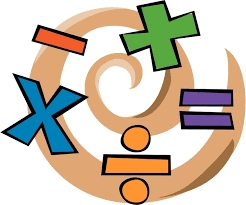We would be pleased if you find an error in the word problem, spelling mistakes, or inaccuracies and send it to us. Thank you!Tips to related online calculators
Need help calculate sum, simplify or multiply fractions? Try our fraction calculator.

## Next similar math problems:

• Here isHere is a data set (n=117) that has been sorted. 10.4 12.2 14.3 15.3 17.1 17.8 18 18.6 19.1 19.9 19.9 20.3 20.6 20.7 20.7 21.2 21.3 22 22.1 22.3 22.8 23 23 23.1 23.5 24.1 24.1 24.4 24.5 24.8 24.9 25.4 25.4 25.5 25.7 25.9 26 26.1 26.2 26.7 26.8 27.5 27.6 2
• Expressions with variableThis is algebra. Let n represent an unknown number and write the following expressions: 1. 4 times the sum of 7 and the number x 2. 4 times 7 plus the number x 3. 7 less than the product of 4 and the number x 4. 7 times the quantity 4 more than the number
• ExpressionsLet k represent an unknown number, express the following expressions: 1. The sum of the number n and two 2. The quotient of the number n and nine 3. Twice the number n 4. The difference between nine and the number n 5. Nine less than the number n
• Turtles 2A box turtle hibernates in the sand at 11 5/8. A spotted turtle hibernates at 11 16/25 feet. Which turtle is deeper?
• DiophantusWe know little about this Greek mathematician from Alexandria, except that he lived around 3rd century A. D. Thanks to an admirer of his, who described his life through an algebraic riddle, we know at least something about his life. Diophantus's youth las
• NumbersDetermine the number of all positive integers less than 4183444 if each is divisible by 29, 7, 17. What is its sum?On the meadow grazing horses, cows and sheep, together less than 200. If cows were 45 times more, horses 60 times more and sheep 35 times more than there are now, their numbers would equall. How many horses, cows and sheep are on the meadow together?
• Speed of Slovakian trainsRudolf decided to take the train from the station 'Ostratice' to 'Horné Ozorovce'. In the train timetables found train Os 5409 : km 0 Chynorany 15:17 5 Ostratice 15:23 15:23 8 Rybany 15:27 15:27 10 Dolné Naštice 15:31 15:31 14 Bánovce nad Bebravou 15:35 1
• Z9–I–4 MO 2017Numbers 1, 2, 3, 4, 5, 6, 7, 8 and 9 were prepared for a train journey with three wagons. They wanted to sit out so that three numbers were seated in each carriage and the largest of each of the three was equal to the sum of the remaining two. The conduct
• Toilets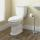A federal law requires that all residential toilets sold in the United States use no more than 1 3/5 gallons of water per flush. Prior to this legislation, conventional toilets used 3 2/5 gallons of water per flush. Find the amount of water saved in one y
• Students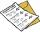The class has 22 students. 2 of which has assesement of 2. Count of assesement of 1 is three times more than count of students with assesement of 3. How many students has assesement of 1 and how many students has assesement of 1.
• Three numbersFind three numbers so that the second number is 4 times greater than the first and the third is lower by 5 than the second number. Their sum is 67.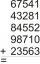Fill letters instead of digits so the indicated sum (equal letters represent equal digits). What number is hidden under the letter J? A A H A H O A H O J -------------------------- 4 3 2 1Solve integer equation: a +b+c =30 a, b, c = can be odd natural number from this set (1,3,5,7,9,11,13,15)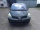Peter plans to buy a used car: the first car Renault Espace 2.0 dCi 16V Dynamique 2006, costs 2000 euros. It is 14 years old and has a combined diesel consumption of 8 liters. / 100 km. Diesel costs 1.1 euros/liter. How much will the car cost him to operaFrom Levíc to Košíc go car at speed 81 km/h. From Košíc to Levíc go another car at speed 69 km/h. How many minutes before the meeting will be cars 27 km away?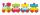The numbers 1,2,3,4,5,6,7,8 and 9 traveled by train. The train had three cars and each was carrying just three numbers. No. 1 rode in the first carriage, and in the last carriage was all odd numbers. The conductor calculated sum of the numbers in the firs